# Introduction

Each statistics and biostatistics department around the world, in conjunction with their collaborators (for us at the University of Washington this includes departments like Epidemiology and Global Health), must choose a statistical software to teach in introductory courses. At the University of Washington, STATA has been taught for many years. While STATA is a powerful software, with relatively user-friendly syntax, it is not very flexible. Only one data set can be read in at a time, and there is only one data type. Simulations are especially difficult to run. While STATA is an important tool to know, we argue that learning the fundamentals of R opens up many possibilities and a lot of power.

We have written another document, “An Introduction to R”, which serves as an introduction to the R philosophy of programming and lays out some of the basic data manipulation strategies. In this document, we assume that the reader has read “An Introduction to R” or is at least familiar with the basic R data types, data manipulation, basic functions for descriptive statistics, installing and loading packages. We now present a detailed walkthrough of functions in R that perform the same task as functions in STATA, with syntax provided.

Many of these functions are available in the base R package, which is automatically installed when R is installed and automatically loaded each time you boot up R. However, some functions are only available in other packages which you have to install and load manually. In particular, one of the goals of the uwIntroStats package is to facilitate easy adoption of R for STATA users. We leave introduction of this package to a separate document entitled “Using the uwIntroStats Package”.

# Descriptive Statistics

## Simple Descriptives

Quick view of functions:

STATA R (base)
summarize summary(), mean(), sd(), var(), min(), max()

In STATA, the summarize command calculates the number of observations, mean, standard deviation, minimum, and maximum value for each variable in the data set. The STATA command conveniently displays all of these summary statistics with one command. In R, this is a bit harder. To find the mean or the median, we simply type mean(data) or median(data). Many of the other simple functions (sum - compute a sum, dim - return the dimensions of an object) operate in a similar way. For our example, let’s use the mri data set. In STATA, we need to download from the internet and read in the file. We can also do this in R (see “An Introduction to R” for more information), but it is much easier and faster to load it in from the uwIntroStats package.

Example 1

Goal: Calculate summary statistics - mean, standard deviation, minimum, and maximum - for variables in the mri data.

Data: mri

STATA Code and Output

* First read in the mri data *
infile ptid mridate age male race weight height packyrs yrsquit alcoh physact chf chd stroke diabetes genhlth ldl alb crt plt sbp aai fev dsst atrophy whgrd numinf volinf obstime death using "http://www.emersonstatistics.com/datasets/mri.txt"

* This will throw ''errors'' - this is due to the headers in the file *
* Delete this first row of NAs *
drop in 1

/* Now call summarize. Note that normally this will print all variables, but we have not included them all */
summarize

Variable |       Obs        Mean    Std. Dev.       Min        Max
-------------+--------------------------------------------------------
ptid |       735         368    212.3205          1        735
mridate |       735    76422.93    31896.42      10192     123191
age |       735    74.56599    5.451364         65         99
male |       735    .4979592    .5003363          0          1
race |       735    1.318367    .6659304          1          4
-------------+--------------------------------------------------------

R Code and Output:

## Load the uwIntroStats package
library(uwIntroStats)
##
## Attaching package: 'uwIntroStats'
##
## The following object is masked from 'package:base':
##
##     tabulate
## Load the mri data
data(mri)
## Calculate summary statistics
mean(mri$age) ##  74.56599 median(mri$age)
##  74
min(mri$age) ##  65 max(mri$age)
##  99
sd(mri$age) ##  5.451364 length(mri$age)
##  735

The R functions give us flexible options in case we ever need only a subset of these summary statistics. If we wanted to apply one of these functions to the entire data, we could use the apply() function (again, we only display the first five elements for the sake of space):

head(apply(mri, 2, mean, na.rm = TRUE), n = 5)
##         ptid      mridate          age         male         race
## 3.680000e+02 7.642293e+04 7.456599e+01 4.979592e-01 1.318367e+00

The last argument, na.rm = TRUE, is passed to the mean() function and makes sure that missing values are removed from the variable when attempting to calculate the mean. Otherwise, the function will return NA.

## The Chi-squared test

Quick view of functions:

STATA R (base)
tabulate, chi2 table(), chisq.test()
cc
cs

In STATA, tabulate displays tables of frequency counts. If you add the oneway or twoway arguments, a chi-squared test is performed as well. The chi-squared test in this case is a descriptive statistic - it measures how well the observations match up with the expected distribution based on the sample proportions. The cc and cs commands also compute chi-squared tests, on case control and cohort data respectively.

The base R function chisq.test() performs the chi-squared test on tables, matrices, and vectors. The function table() returns a frequency table of the variables entered. However, in order to calculate Odds Ratios, Risk Ratios, or other statistics (for example the likelihood ratio, Mantel-Haenszel statistic, and others) you must use other functions developed for different R packages and piece the information together. For example, the Exact package and the uwIntroStats package both have functions for calculating these ratios. These packages give more output than the base R functions, and in the case of uwIntroStats follow a similar syntax to STATA.

Example 1

Goal: Calculate chi-squared statistics on the tabulation of male and diabetes.

Data: mri STATA Code and Output

cc male diabetes
Proportion
|   Exposed   Unexposed  |      Total     Exposed
-----------------+------------------------+------------------------
Cases |        53         313  |        366       0.1448
Controls |        26         343  |        369       0.0705
-----------------+------------------------+------------------------
Total |        79         656  |        735       0.1075
|                        |
|      Point estimate    |    [95\% Conf. Interval]
|------------------------+------------------------
Odds ratio |         2.233841       |    1.333028    3.814119 (exact)
Attr. frac. ex. |         .5523406       |    .2498281    .7378163 (exact)
Attr. frac. pop |         .0799837       |
+-------------------------------------------------
chi2(1) =    10.59  Pr>chi2 = 0.0011

cs male diabetes

| diabetes               |
|   Exposed   Unexposed  |      Total
-----------------+------------------------+------------
Cases |        53         313  |        366
Noncases |        26         343  |        369
-----------------+------------------------+------------
Total |        79         656  |        735
|                        |
Risk |  .6708861    .4771341  |   .4979592
|                        |
|      Point estimate    |    [95\% Conf. Interval]
|------------------------+------------------------
Risk difference |         .1937519       |    .0833099     .304194
Risk ratio |         1.406074       |    1.181535    1.673285
Attr. frac. ex. |            .2888       |    .1536436    .4023731
Attr. frac. pop |         .0418208       |
+-------------------------------------------------
chi2(1) =    10.59  Pr>chi2 = 0.0011


R Code and Output

table(mri$male, mri$diabetes)
##
##       0   1
##   0 343  26
##   1 313  53
## Use the "correct = FALSE" argument to halt application of continuity correction
chisq.test(mri$male, mri$diabetes, correct = FALSE)
##
##  Pearson's Chi-squared test
##
## data:  mri$male and mri$diabetes
## X-squared = 10.588, df = 1, p-value = 0.001138

# Plots

## Boxplots

Quick view of functions:

STATA R (base)
graph box boxplot()

The boxplot is usually used as a method of visualizing data quickly, since it reduces the data down to essentially four numbers: minimum, maximum, median, and interquartile range. The syntax for boxplots is quite similar between STATA and R, and each gives approximately the same output. However, it is important to read the documentation for each function to see how outliers’’ are computed. In both cases, the default rule for detecting outliers is those observations which lie outside of 1.5 times the IQR. Knowing the rule is important for deciding whether to classify these observations as outliers or not.

Example 1

Goal: Produce a boxplot to describe the distribution of forced exhalation volume (FEV) by smoking group.

Data: fev

STATA Code and Output

* Read in the data *
use "https://courses.washington.edu/b511/Data/FEV1ClinTrial.dta"

* Rename the variables *
rename Y0 FEV1base
rename Y1 FEV1wk24
rename T smoke

* Make the boxplot *
graph box FEV1base, by(smoke) ytitle(FEV)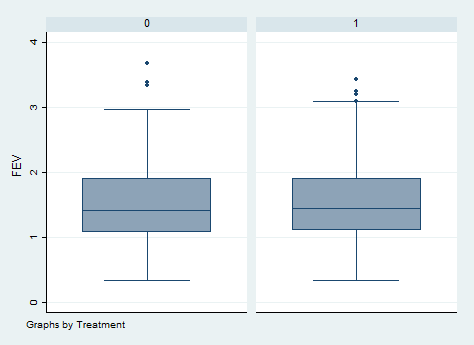R Code and Output

## Read in the data
fev <- read.table("http://courses.washington.edu/b511/Data/FEV1ClinTrial.dat", sep = "")

## Rename the data
names(fev) <- c("FEV1wk24", "FEV1base", "smoke")

## Create the boxplot
boxplot(fev$smoke, fev$FEV1base)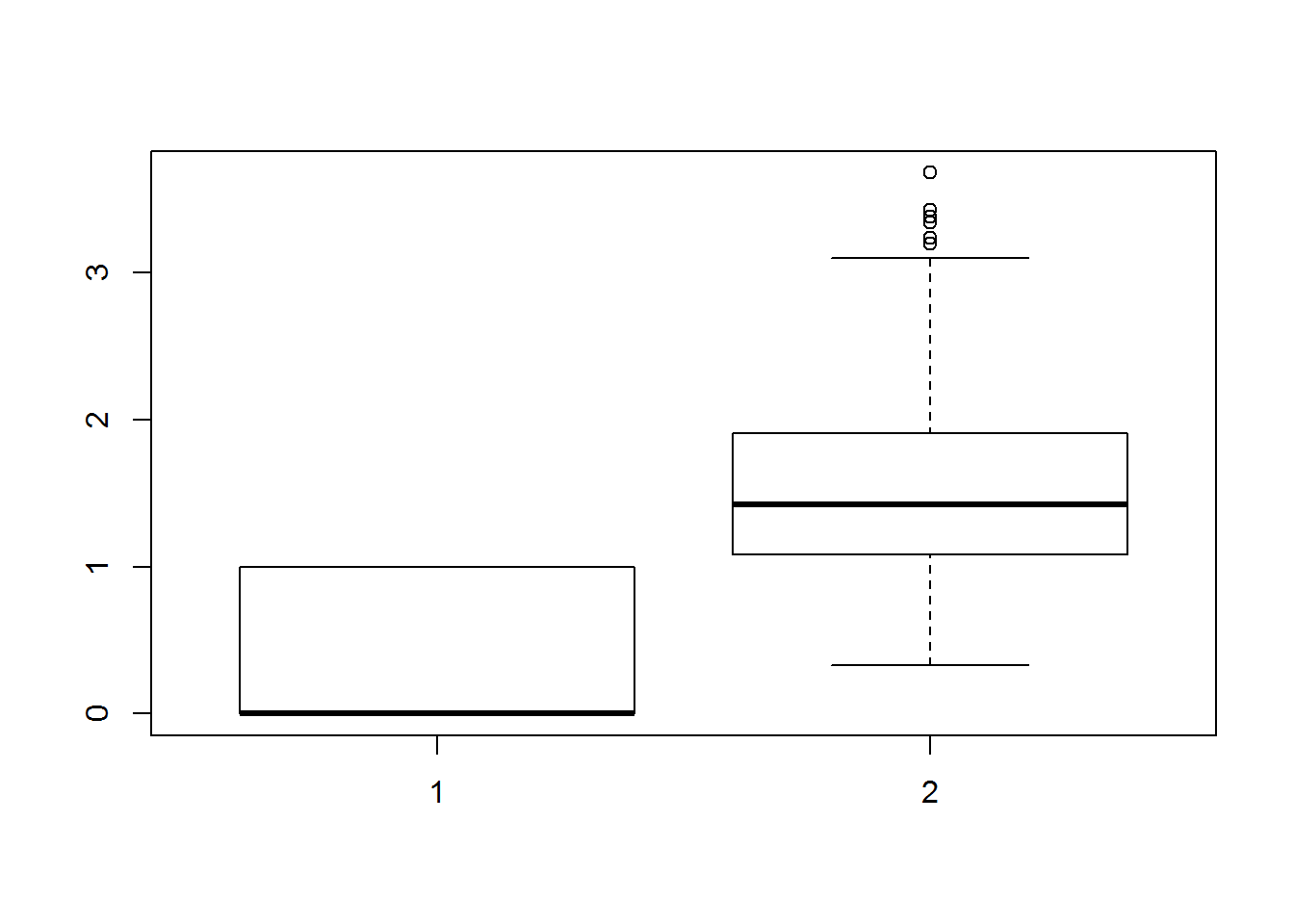## Histograms

Quick view of functions:

STATA R (base)
histogram hist()

The syntax for creating a histogram is very similar between R and STATA. However, if the STATA dataset has labels for the variables, it will automatically put in these labels on the plot. In R, we have to manually label the plot.

Example 1

Goal: Produce a histogram of FEV at week 24.

Data: fev

STATA Code and Output

* Read in the data, rename variables *
use "https://courses.washington.edu/b511/Data/FEV1ClinTrial.dta"
rename Y0 FEV1base
rename Y1 FEV1wk24
rename T smoke

* Create the histogram *
histogram FEV1wk24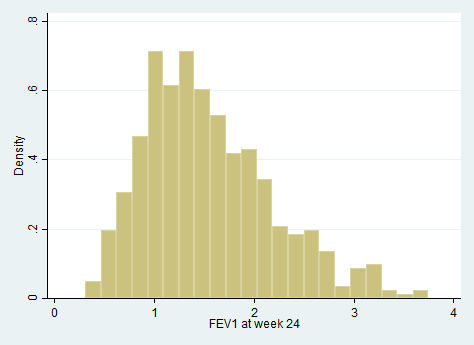R Code and Output

## Read in the data, rename the variables
fev <- read.table("http://courses.washington.edu/b511/Data/FEV1ClinTrial.dat", sep = "")
names(fev) <- c("FEV1wk24", "FEV1base", "smoke")

## Create the histogram
hist(fev$FEV1wk24, xlab = "FEV1 at week 24", main = "Histogram of FEV1 at week 24")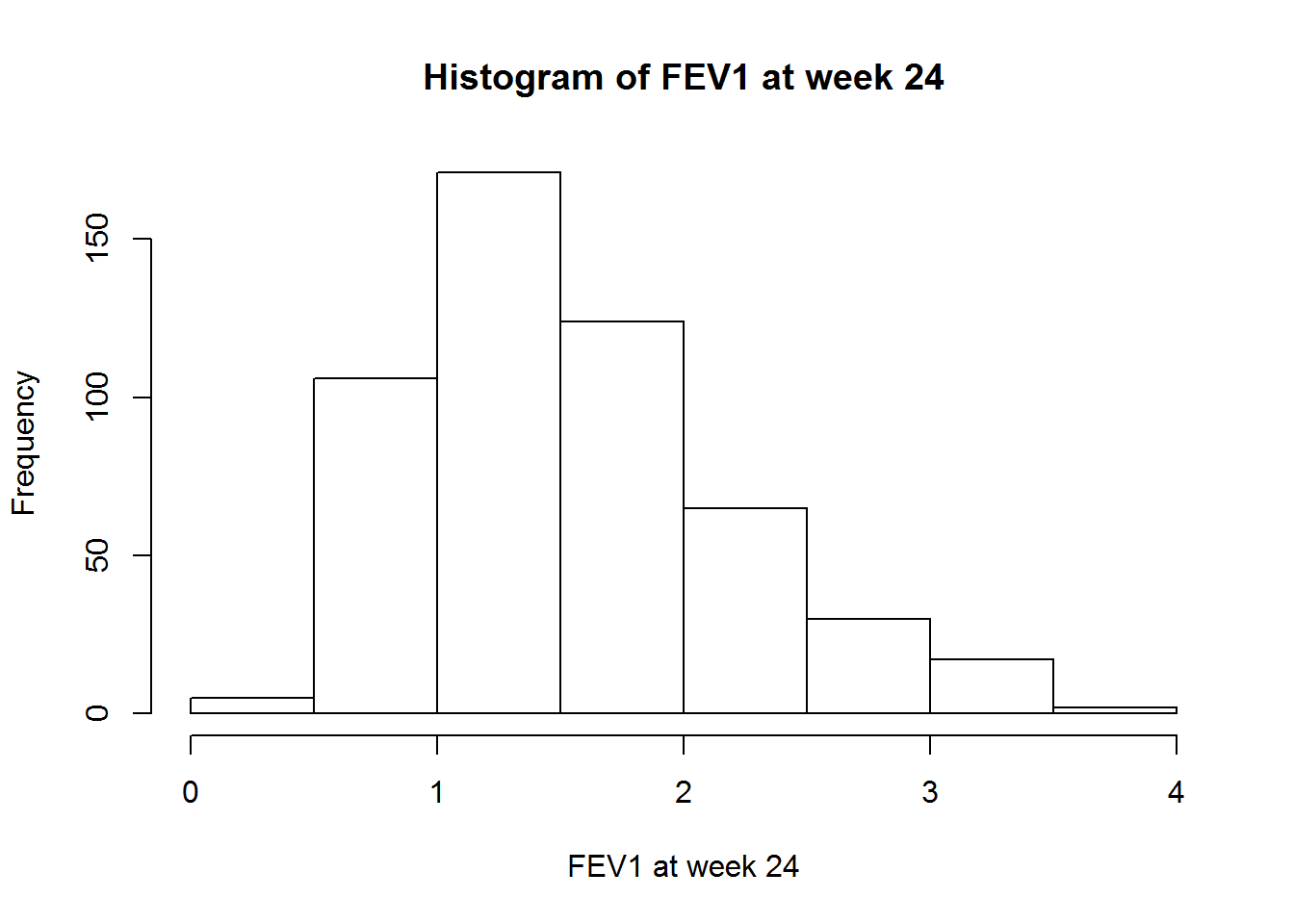## Scatterplots Quick view of functions: STATA R (base) scatter plot scatter.smooth() The base R version of the scatterplot takes an x anda y variable (which must be of the same length) and plots the points as specified by these coordinates. If you wish to have least squares lines or other additions to the plot, you must use the abline() function, or use the scatter.smooth() function. The STATA version of scatterplot takes a y-variable followed by a x-variable, followed by any arguments. Example 1 Goal: Produce a scatterplot of FEV at 24 weeks against FEV at baseline, with loess and least squares lines fit to the graph. Data: fev STATA Code and Output * Read in the data, rename variables * use "https://courses.washington.edu/b511/Data/FEV1ClinTrial.dta" rename Y0 FEV1base rename Y1 FEV1wk24 rename T smoke * Create the scatterplot * scatter FEV1wk24 FEV1base || lfit FEV1wk24 FEV1base || lowess FEV1wk24 FEV1base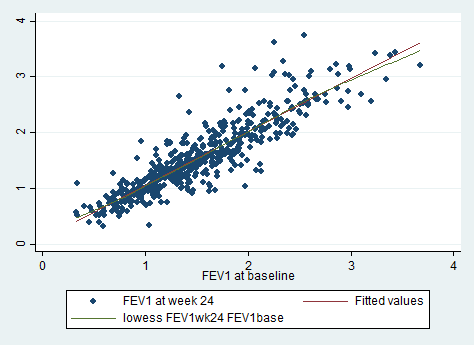R Code and Output ## Read in the data, rename the variables fev <- read.table("http://courses.washington.edu/b511/Data/FEV1ClinTrial.dat", sep = "") names(fev) <- c("FEV1wk24", "FEV1base", "smoke") ## create the scatterplot - this doesn't have loess curve! plot(fev$FEV1base, fev$FEV1wk24, xlab = "FEV1 at baseline", ylab = "FEV1 at week 24", main = "Scatterplot of FEV1 at baseline vs week 24")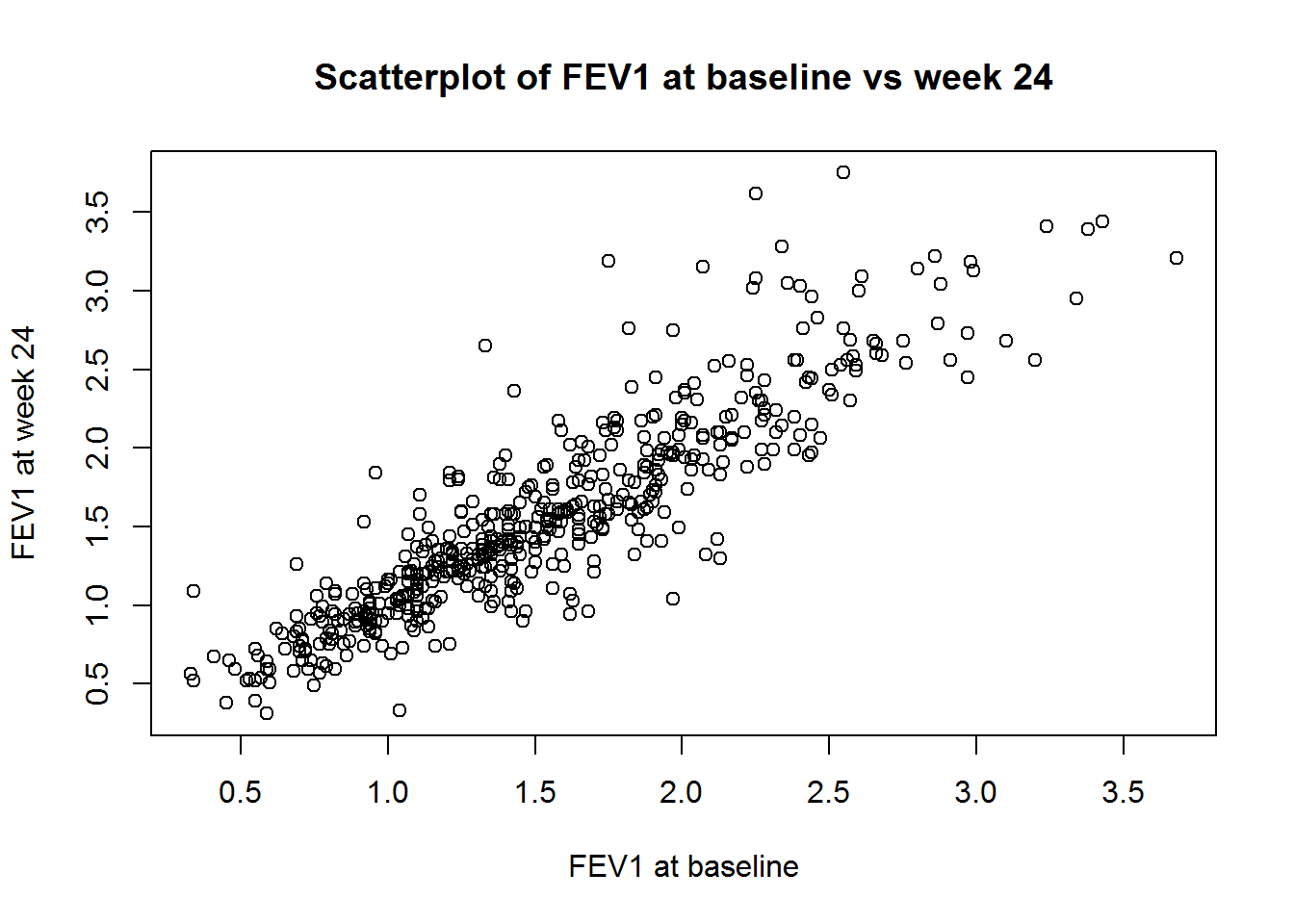scatter.smooth(fev$FEV1wk24, fev$FEV1base, xlab = "FEV1 at baseline", ylab = "FEV1 at week 24", main = "Scatterplot of FEV1 at baseline vs week 24") ## add the least squares line - don't worry about how we calculated the lines for now! fev1.lm <- lm(FEV1wk24 ~ FEV1base, data = fev) abline(fev1.lm, col = "red") ## add a legend legend(.5, 3.5, legend = c("Loess", "Least Squares"), fill = c("black", "red"))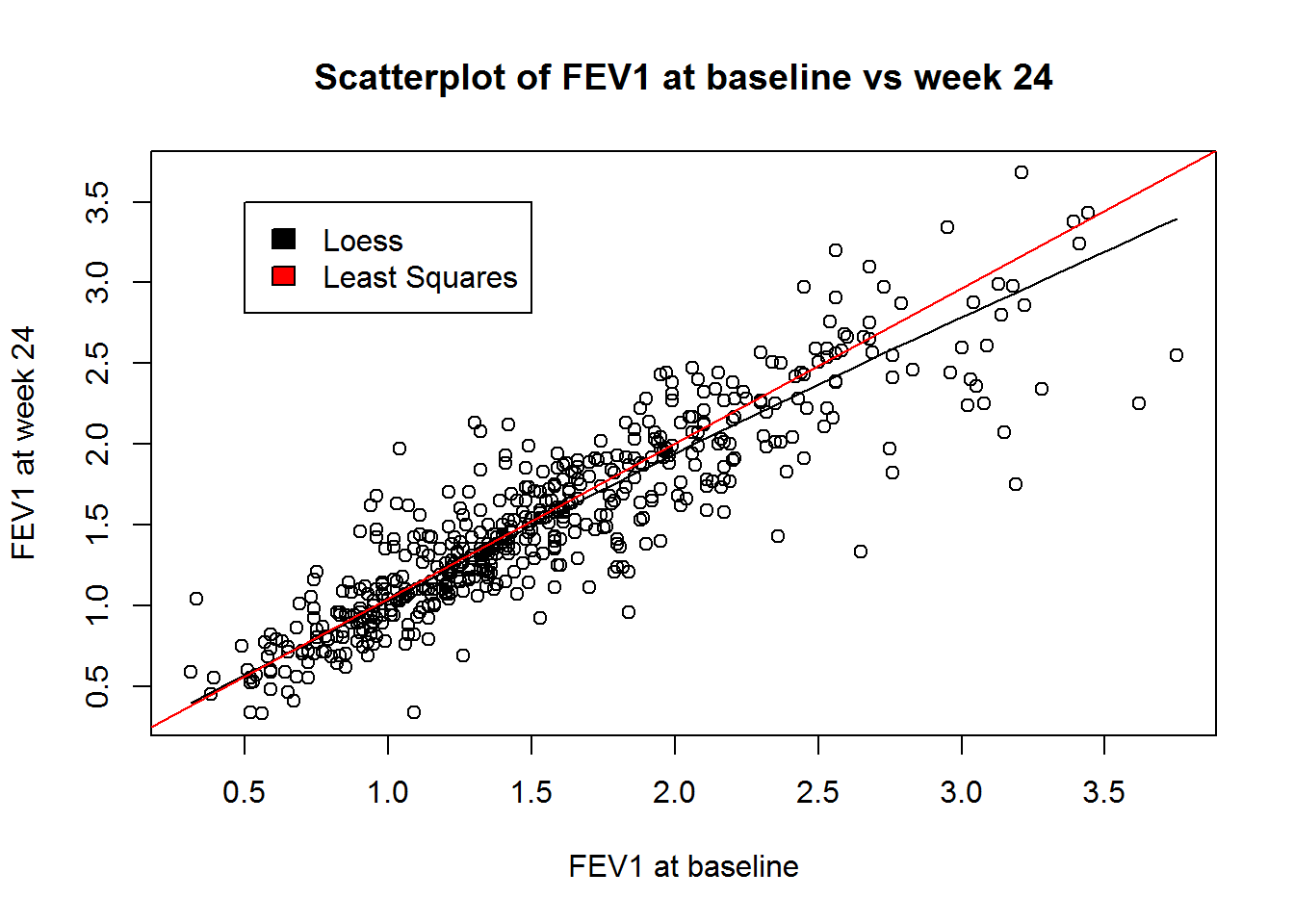## Correlation Quick view of functions: STATA R (base) correlate cor() cov() In STATA, the command to compute a correlation matrix is correlate. In base R, there are a few different functions to compute correlation, covariance, or variance. The cor() function computes the correlation between two vectors or matrices. The cov() function computes the covariance between two vectors or matrices. In both of these cases, if a matrix is entered, then the covariance (or correlation) is computed between the columns of the matrices. Last, var() computes variance as we discussed above, but var() computed on two matrices returns the same as cov(). Example 1 Goal: Compute the correlation and covariance between FEV at week 24 and FEV at baseline. Data: fev STATA Code and Output * Read in the data, rename variables * use "https://courses.washington.edu/b511/Data/FEV1ClinTrial.dta" rename Y0 FEV1base rename Y1 FEV1wk24 rename T smoke * Compute * correlate FEV1wk24 FEV1base (obs=520) | FEV1wk24 FEV1base -------------+------------------ FEV1wk24 | 1.0000 FEV1base | 0.8932 1.0000 correlate FEV1wk24 FEV1base, cov (obs=520) | FEV1wk24 FEV1base -------------+------------------ FEV1wk24 | .413977 FEV1base | .343671 .357618 Notice that the STATA output when we asked for the covariances gave the covariance in the lower left, and the variance of the individual variables along the diagonal. R Code and Output ## Read in the data, rename variables fev <- read.table("http://courses.washington.edu/b511/Data/FEV1ClinTrial.dat", sep = "") names(fev) <- c("FEV1wk24", "FEV1base", "smoke") ## Compute cor(fev$FEV1wk24, fev$FEV1base) ##  0.8931929 cov(fev$FEV1wk24, fev$FEV1base) ##  0.3436712 var(fev$FEV1wk24)
##  0.413977
var(fev$FEV1base) ##  0.3576181 To run pairwise correlations, in R you need to add the argument use = "pairwise.complete.obs". To run a different method of correlation calculation, use the method argument, and set it to either "pearson" (default), "spearman", or "kendall". In STATA, use the pwcorr command, which has all of the same arguments as the correlate command. # Distribution Functions Quick view of functions: STATA R (base) display distnameprob(statistic) pdistname(statistic) Say we calculate a test statistic (z-score, t-statistic, chi-squared statistic) and want to find the p-value. Recall that for a p-value we want to find the probability of values at least as extreme as our value, given that the null hypothesis is true. If we calculate a z-score of 1 for our data, then we know that we are dealing with the normal distribution. To find the p-value, then, we have to call the cumulative distribution function command in R or in STATA. Example 1 has the correct code for this example. The other distributions both follow the convention in this example, and for that reason we have placed distname in italics in the “Quick view” section. Example 1 Goal: Calculate the p-value associated with a z-score of 1. Data: None. STATA Code and Output display 1-normprob(1) .15865525 R Code and Output 1 - pnorm(1) ##  0.1586553 # One Sample Inference ## The One Sample t-test Quick view of functions: STATA R (base) ttest t.test() While the commands for the t-test look very similar in STATA and in R, there are a few differences. Most noticeably, the output is very different. Also, STATA provides inference for each one-sided hypothesis (both that the mean is less than the null hypothesized value, and that it is greater) individually, while R defaults to providing inference for the two-sided test. Using the alternative argument to t.test()allows the user to change the inference by providing either "less" or "greater" for a one-sided test. Example 1 Goal: Test that the mean atrophy score is equal to 30. Data: mri STATA Code and Output ttest atrophy == 30 One-sample t test ------------------------------------------------------------------------------ Variable | Obs Mean Std. Err. Std. Dev. [95% Conf. Interval] ---------+-------------------------------------------------------------------- atrophy | 735 35.98367 .4766699 12.92294 35.04787 36.91947 ------------------------------------------------------------------------------ mean = mean(atrophy) t = 12.5531 Ho: mean = 30 degrees of freedom = 734 Ha: mean < 30 Ha: mean != 30 Ha: mean > 30 Pr(T < t) = 1.0000 Pr(|T| > |t|) = 0.0000 Pr(T > t) = 0.0000 R Code and Output ## Two-sided test t.test(mri$atrophy, mu = 0)
##
##  One Sample t-test
##
## data:  mri$atrophy ## t = 75.49, df = 734, p-value < 2.2e-16 ## alternative hypothesis: true mean is not equal to 0 ## 95 percent confidence interval: ## 35.04787 36.91947 ## sample estimates: ## mean of x ## 35.98367 ## One-sided tests t.test(mri$atrophy, mu = 0, alternative = "less")
##
##  One Sample t-test
##
## data:  mri$atrophy ## t = 75.49, df = 734, p-value = 1 ## alternative hypothesis: true mean is less than 0 ## 95 percent confidence interval: ## -Inf 36.76872 ## sample estimates: ## mean of x ## 35.98367 t.test(mri$atrophy, mu = 0, alternative = "greater")
##
##  One Sample t-test
##
## data:  mri$atrophy ## t = 75.49, df = 734, p-value < 2.2e-16 ## alternative hypothesis: true mean is greater than 0 ## 95 percent confidence interval: ## 35.19863 Inf ## sample estimates: ## mean of x ## 35.98367 # Two Sample Inference ## The Two Sample t-test Quick view of functions: STATA R (base) ttest t.test() Both the ttest command in STATA and the t.test() function in R are equipped to handle two-sample inference as well. Both functions by default presume that the two groups have equal variances. They also provide the option that the test is a matched-pairs test. Example 1 Goal: Test whether the mean atrophy score is different between males and females. Data: mri STATA Code and Output ttest atrophy, by(male) Two-sample t test with equal variances ------------------------------------------------------------------------------ Group | Obs Mean Std. Err. Std. Dev. [95% Conf. Interval] ---------+-------------------------------------------------------------------- 0 | 369 32.90515 .6363956 12.22476 31.65372 34.15658 1 | 366 39.08743 .6733026 12.88104 37.76339 40.41147 ---------+-------------------------------------------------------------------- combined | 735 35.98367 .4766699 12.92294 35.04787 36.91947 ---------+-------------------------------------------------------------------- diff | -6.182283 .9262663 -8.000734 -4.363831 ------------------------------------------------------------------------------ diff = mean(0) - mean(1) t = -6.6744 Ho: diff = 0 degrees of freedom = 733 Ha: diff < 0 Ha: diff != 0 Ha: diff > 0 Pr(T < t) = 0.0000 Pr(|T| > |t|) = 0.0000 Pr(T > t) = 1.0000 R Code and Output t.test(mri$atrophy[mri$male == 0], mri$atrophy[mri$male == 1]) ## ## Welch Two Sample t-test ## ## data: mri$atrophy[mri$male == 0] and mri$atrophy[mri\$male == 1]
## t = -6.673, df = 730.33, p-value = 4.956e-11
## alternative hypothesis: true difference in means is not equal to 0
## 95 percent confidence interval:
##  -8.001133 -4.363432
## sample estimates:
## mean of x mean of y
##  32.90515  39.08743

The t-test in R requires that we subset our data if we want to test within another group.

# Linear Regression

Quick view of functions:

STATA R (base)
regress lm()

In STATA, the regress command takes the dependent variable (y) followed by the independent variables (x). In R, we need to give lm() a formula - an object with a “~”" character. For example, if we wanted to regress atrophy on age in the mri data, the formula would be

atrophy ~ age

We also need to supply lm() with a dataset, using the data = argument.

The call to lm() creates a lm object, and in order to print the full inference (like STATA does by default) we need to use the summary() function. Once we have done this, the output agrees.

Example 1

Goal: Determine the association between age and cerebral atrophy by regressing atrophy on age.

Data: mri

STATA Code and Output

regress atrophy age

Source |       SS       df       MS              Number of obs =     735
-------------+------------------------------           F(  1,   733) =   69.58
Model |   10626.648     1   10626.648           Prob > F      =  0.0000
Residual |  111953.156   733  152.732819           R-squared     =  0.0867
Total |  122579.804   734  167.002458           Root MSE      =  12.359

------------------------------------------------------------------------------
atrophy |      Coef.   Std. Err.      t    P>|t|     [95% Conf. Interval]
-------------+----------------------------------------------------------------
age |   .6979831   .0836783     8.34   0.000     .5337054    .8622609
_cons |  -16.06213   6.256186    -2.57   0.010    -28.34431   -3.779947
------------------------------------------------------------------------------

R Code and Output

atr.lm <- lm(atrophy ~ age, data = mri)
summary(atr.lm)
##
## Call:
## lm(formula = atrophy ~ age, data = mri)
##
## Residuals:
##     Min      1Q  Median      3Q     Max
## -36.870  -8.589  -0.870   7.666  51.203
##
## Coefficients:
##              Estimate Std. Error t value Pr(>|t|)
## (Intercept) -16.06213    6.25619  -2.567   0.0104 *
## age           0.69798    0.08368   8.341 3.64e-16 ***
## ---
## Signif. codes:  0 '***' 0.001 '**' 0.01 '*' 0.05 '.' 0.1 ' ' 1
##
## Residual standard error: 12.36 on 733 degrees of freedom
## Multiple R-squared:  0.08669,    Adjusted R-squared:  0.08545
## F-statistic: 69.58 on 1 and 733 DF,  p-value: 3.635e-16

## Generalized Linear Models

Quick view of functions:

STATA R (base)
glm, family() link() glm(family = )
stcox coxph()

Generalized linear models allow the user to perform logistic regression, poisson regression, and proportional hazards regression, to name a few. In both STATA and R, for all of the types besides proportional hazard regression, the function is glm. In these cases, we need to supply the function with a family so that the correct type of regression is run. We also sometimes need to specify the correct link function. The link function specifies what function of the parameter of interest (usually mean response) is linear in the coefficients. For example, in logistic regression, we use a log link function because our assumption is that (for a model with only one predictor): $\log (E(Y)) = \beta_0 + \beta_1 X$ In both cases, binomial is the correct family for logistic regression. However, in STATA, we need to specify a link function to differentiate logistic (link(logit)) from poisson (link(probit)) regression. In R, we use family = "poisson" to run poisson regression.

In both languages, proportional hazards regression requires a special function. In STATA this is stcox, while in R it is coxph(). Both of these functions have the same syntax as the functions for linear regression in their respective languages.

Example 1

Goal: Examine the relationship between diabetes and age using logistic regression.

Data: mri

STATA Code and Output

glm diabetes age, family(binomial) link(log)

Iteration 0:   log likelihood = -347.07514
Iteration 1:   log likelihood = -251.86635
Iteration 2:   log likelihood = -250.64888
Iteration 3:   log likelihood = -250.64853
Iteration 4:   log likelihood = -250.64853

Generalized linear models                          No. of obs      =       735
Optimization     : ML                              Residual df     =       733
Scale parameter =         1
Deviance         =  501.2970586                    (1/df) Deviance =  .6838978
Pearson          =  734.9984355                    (1/df) Pearson  =  1.002726

Variance function: V(u) = u*(1-u)                  [Bernoulli]
Link function    : g(u) = ln(u)                    [Log]

AIC             =   .687479
Log likelihood   = -250.6485293                    BIC             = -4336.408

------------------------------------------------------------------------------
|                 OIM
diabetes |      Coef.   Std. Err.      z    P>|z|     [95% Conf. Interval]
-------------+----------------------------------------------------------------
age |  -.0108457   .0201345    -0.54   0.590    -.0503086    .0286172
_cons |  -1.423417   1.498109    -0.95   0.342    -4.359657    1.512823
------------------------------------------------------------------------------


R Code and Output

diabetes.glm <- glm(diabetes ~ age, data = mri, family = "binomial")
summary(diabetes.glm)
##
## Call:
## glm(formula = diabetes ~ age, family = "binomial", data = mri)
##
## Deviance Residuals:
##     Min       1Q   Median       3Q      Max
## -0.5032  -0.4863  -0.4780  -0.4619   2.2011
##
## Coefficients:
##             Estimate Std. Error z value Pr(>|z|)
## (Intercept) -1.21557    1.66965  -0.728    0.467
## age         -0.01211    0.02242  -0.540    0.589
##
## (Dispersion parameter for binomial family taken to be 1)
##
##     Null deviance: 501.59  on 734  degrees of freedom
## Residual deviance: 501.30  on 733  degrees of freedom
## AIC: 505.3
##
## Number of Fisher Scoring iterations: 4

# Post-Estimation

Quick view of functions:

STATA R (base)
lincom
predict predict()

Post-estimation - for the most part - deals with analyzing combinations of coefficients or predicting new values. Once you have run a regression analysis, these functions can supply additional insight into the relationship of interest.

In STATA, the main command for analyzing combinations of coefficients is lincom. This command computes point estimates, t-statistics, confidence intervals, and p-values for the analysis of a linear combination of the regression coefficients against some null hypothesis. For example, if we were exploring the relationship between atrophy, age, and sex in the mri data, we could test if the coefficients for age and male summed to zero. In base R there is no function to do this. However, in the uwIntroStats package, the lincom() function does this.

Example 1

Goal: Using the results from a linear regression model modeling atrophy on age and male, test whether the coefficient estimates of these two variables sum to zero.

Data: mri

STATA Code and Output

lincom age+male

( 1)  age + male = 0

------------------------------------------------------------------------------
atrophy |      Coef.   Std. Err.      t    P>|t|     [95% Conf. Interval]
-------------+----------------------------------------------------------------
(1) |   6.645784   .8870476     7.49   0.000     4.904324    8.387245
------------------------------------------------------------------------------


R Code and Output

Next, suppose we wish to predict the atrophy score for a new participant who is 70 years old and female. We need to use the predict command in STATA or the predict() function in base R. Regardless of the type of regression we have run, both of these functions will work.

Example 2

Goal: Using the results from a linear regression model modeling atrophy on age and male, predict the atrophy score of a 70 year old female.

Data: mri

STATA Code and Output

predict preds
list preds in 1/10

Now we have to match the correct predictions to the age and sex combinations, which is a bit tedious. R Code and Output

reg <- regress("mean", atrophy ~ age + male, data = mri)
newdata <- c(70, 0)
newdata <- as.data.frame(newdata)
## we need to switch the rows and columns to make this work
newdata <- as.data.frame(t(newdata))
colnames(newdata) <- c("age", "male")
predict(reg, newdata)
##  newdata
## 29.90039

While we have had to do some extra work in R to create a data frame, once we have this data frame the prediction is quite simple. As is the case in STATA, the new data must have the same column names as the variables used to build the regression model.